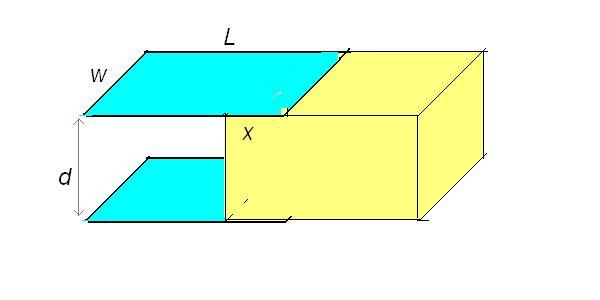# Capacitor and dielectric

Patrikp
• 1. The problem statement,
Dielectric plate is placed in capacitor to the half(picture) and it can freely move. Capacitor consists of two parallel plates and is isolated. Consider two cases:
a)charges on plates are constant,
b)voltage is constant.
What happens in each case?

2. Homework Equations

$$W=\frac{1}{2}CV^2=\frac{q^2}{2C}$$
$$F=\frac{dW}{dx}\\ \text{(1)}$$
$$C=εεr \frac{A}{d}$$

## The Attempt at a Solution

I know that the answer is that the dielectric will be moving inward in both cases and I even have written solution but I don't understand it. I guess that I should find force on dielectric from (2) but before that i need to determine C which I don't know how to do since dielectric is in wierd position.[/B]

#### Attachments

•image.jpg
47.2 KB · Views: 421
Last edited: